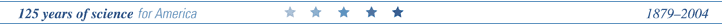U.S. GEOLOGICAL SURVEY
Scientific Investigations Report 2004&ndash;5237

## Conversion Factors and Datums

Multiply By To obtain
Length
inch (in.) 2.54 centimeter
inch (in.) 25.4 millimeter
foot (ft) .3048 meter
mile (mi) 1.609 kilometer
Area
acre 4,047 square meter
acre .4047 hectare
acre .004047 square kilometer
square foot (ft2) 929.0 square centimeter
square foot (ft2) .09290 square meter
section (640 acres or 1 square mile) 259.0 square hectometer
square mile (mi2) 259.0 hectare
square mile (mi2) 2.590 square kilometer
Volume
gallon (gal) 3.785 liter
gallon (gal) .003785 cubic meter
million gallons (Mgal) 3,785 cubic meter
cubic foot (ft3) .02832 cubic meter
acre-foot (acre-ft) 1,233 cubic meter
acre-foot (acre-ft) .001233 cubic hectometer
Flow rate
acre-foot per year (acre-ft/yr) 1,233 cubic meter per year
acre-foot per year (acre-ft/yr) .001233 cubic hectometer per year
foot per day (ft/d) .3048 meter per day
cubic foot per second (ft3/s) .02832 cubic meter per second
gallon per day (gal/d) .003785 cubic meter per day
million gallons per day (Mgal/d) .04381 cubic meter per second
inch per year (in/yr) 25.4 millimeter per year
Hydraulic conductivity
foot per day (ft/d) 0.3048 meter per day
foot per mile (ft/mi) 0.1894 meter per kilometer
Transmissivity*
foot squared per day (ft2/d) 0.09290 meter squared per day

Temperature in degrees Celsius (°C) may be converted to degrees Fahrenheit (°F) as follows:

°F=(1.8×°C+32).

Temperature in degrees Fahrenheit (°F) may be converted to degrees Celsius (°C) as follows:

°C=(°F-32/1.8).

*Transmissivity: The standard unit for transmissivity is cubic foot per day per square foot times foot of aquifer thickness [(ft3/d/ft2]ft. In this report, the mathematically reduced form, foot squared per day (ft2/d), is used for convenience.

### DATUMS

Vertical coordinate information is referenced to the National Geodetic Vertical Datum of 1929 (NGVD29).

Horizontal coordinate information is referenced to the North American Datum of 1983 (NAD83).

Altitude, as used in this report, refers to distance above or below sea level.